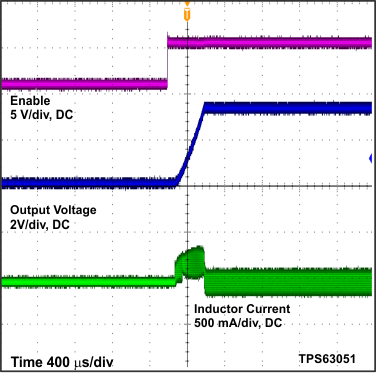SLVSAM8D July   2013  – August 2019

PRODUCTION DATA.

1. Features
2. Applications
3. Description
1.     Device Images
4. Revision History
5. Device Comparison Table
6. Pin Configuration and Functions
7. Specifications
8. Detailed Description
1. 8.1 Overview
2. 8.2 Functional Block Diagrams
3. 8.3 Feature Description
4. 8.4 Device Functional Modes
9. Application and Implementation
1. 9.1 Application Information
2. 9.2 Typical Application
1. 9.2.1 Design Requirements
2. 9.2.2 Detailed Design Procedure
3. 9.2.3 Application Curves
10. 10Power Supply Recommendations
11. 11Layout
12. 12Device and Documentation Support
13. 13Mechanical, Packaging, and Orderable Information

• RMW|12
• YFF|12

#### 9.2.3 Application Curves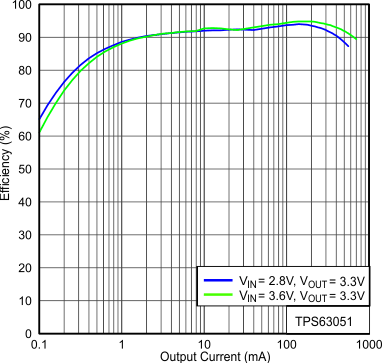PFM/PWM = Low VOUT = 3.3 V
Figure 10. Efficiency vs Output Current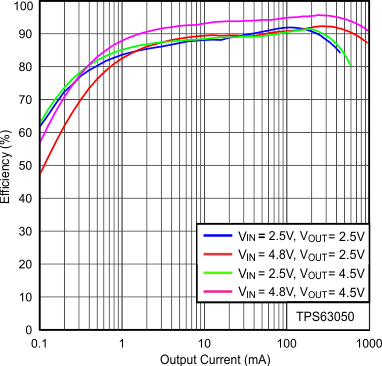PFM/PWM = Low VOUT = 2.5 V, 4.5 V
Figure 12. Efficiency vs Output Current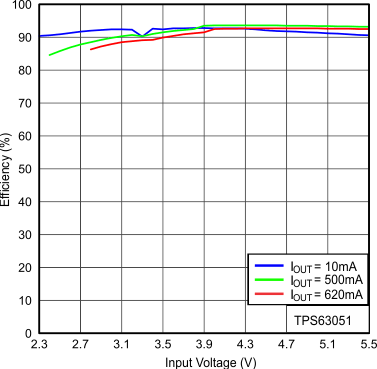PFM/PWM = Low VOUT= 3.3 V
Figure 14. Efficiency vs Input Voltage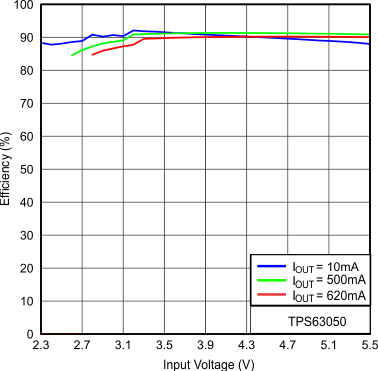PFM/PWM = Low VOUT = 2.5 V
Figure 16. Efficiency vs Input Voltage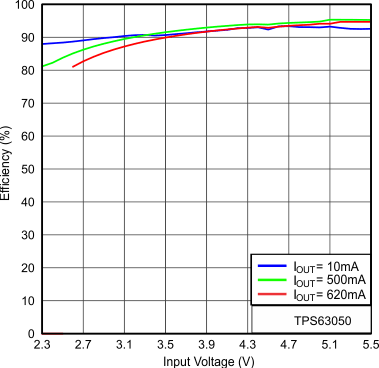PFM/PWM = Low VOUT = 4.5 V
Figure 18. Efficiency vs Input VoltageVIN = 2.5 V
Figure 20. Output Voltage vs Output Current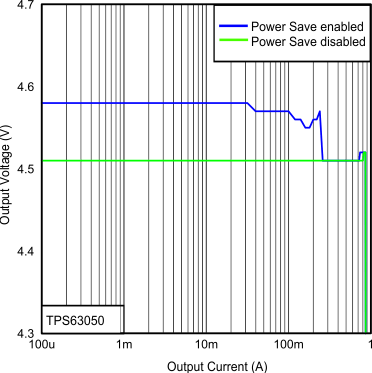VIN = 4.5 V
Figure 22. Output Voltage vs Output Current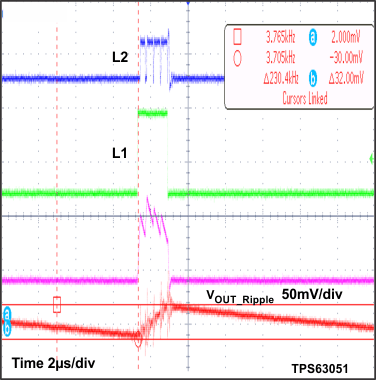VIN = 2.8 V IOUT = 16 mA
Figure 24. Output Voltage Ripple in Boost Mode and PFM to PWM Transition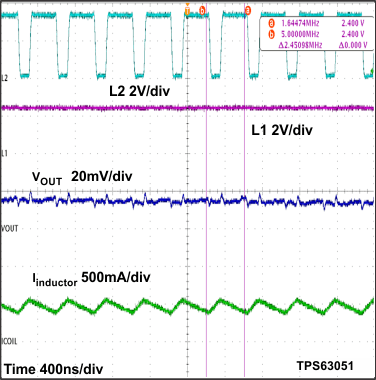VIN = 2.5 V IOUT = 300 mA
Figure 26. Switching Waveform in Boost Mode and PWM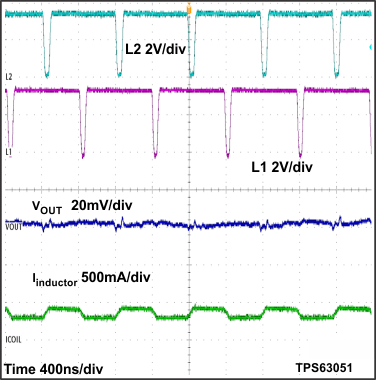VIN = 3.4 V IOUT = 300 mA
Figure 28. Switching Waveform in Buck-Boost Mode and PWM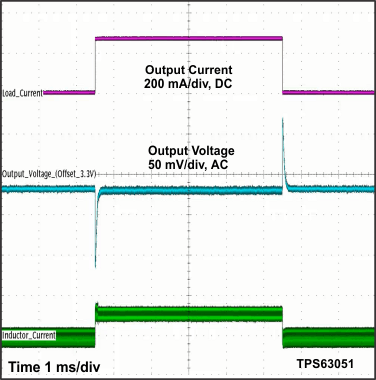VIN = 3.6 V Load change from 0 mA to 300 mAVOUT = 3.3 V VIN = 2.5 V IOUT = 0 mA
Figure 32. Start Up After Enable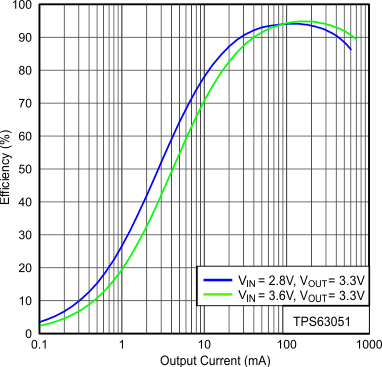PFM/PWM = High VOUT = 3.3 V
Figure 11. Efficiency vs Output Current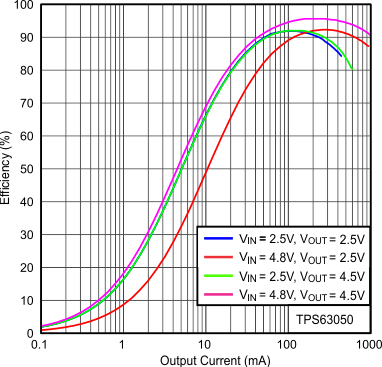PFM/PWM = High VOUT = 2.5 V, 4.5 V
Figure 13. Efficiency vs Output Current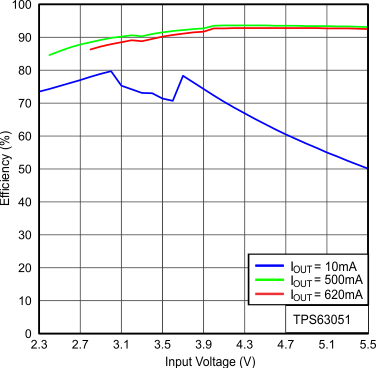PFM/PWM = High VOUT= 3.3 V
Figure 15. Efficiency vs Input Voltage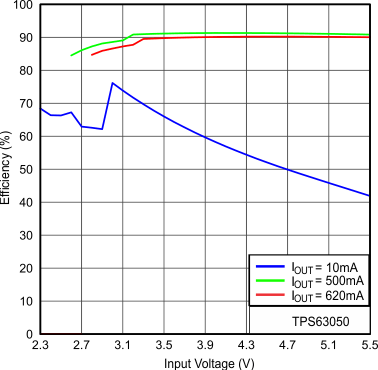PFM/PWM = High VOUT = 2.5 V
Figure 17. Efficiency vs Input Voltage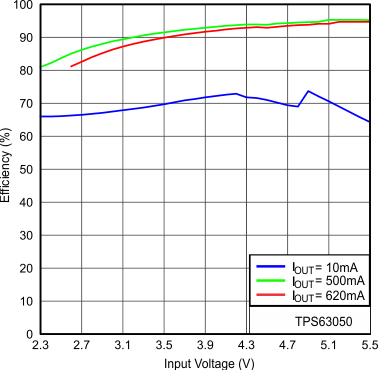PFM/PWM =High VOUT= 4.5 V
Figure 19. Efficiency vs Input VoltageVIN = 3.3 V
Figure 21. Output Voltage vs Output Current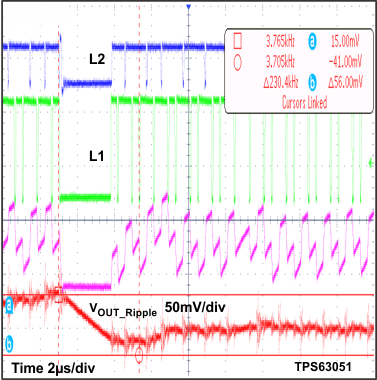VIN = 3.3 V IOUT = 145 mA
Figure 23. Output Voltage ripple in Buck-Boost mode and PFM to PWM transition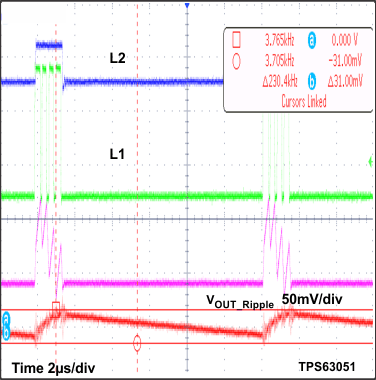VIN = 4.2 V IOUT = 16 mA
Figure 25. Output Voltage Ripple in Buck Mode and PFM to PWM Transition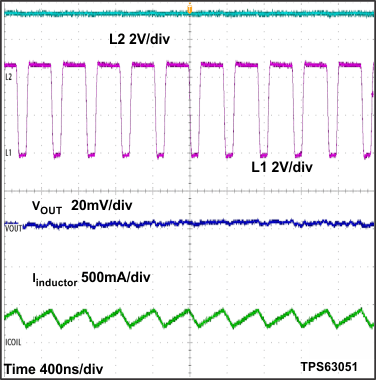VIN = 4.5 V IOUT = 300 mA
Figure 27. Switching Waveform in Buck Mode and PWM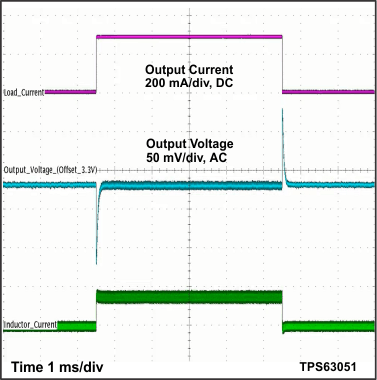VIN = 2.8 V Load change from 0 mA to 300 mA